November, 2016: A slight simplification of the generalized Cauchy-Binet theorem has appeared in the Monthly by Alan Hoffman and Chai Wah Wu. It is essentially the same proof but what is nice that the language of compound matrices avoids exterior powers. The r'th compound matrix is the r'th exterior power. The article A Simple Proof of a Generalized Cauchy-Binet Theorem, by Alan J. Hoffman and Chai Wah Wu The American Mathematical Monthly, Vol. 123, No. 9 (November 2016), pp. 928-930. February 20, 2016: Marvin David Marcus (July 31, 1927-February 20, 2016), one of the experts in linear algebra passed away. The Marcus Minc book is especially amazing. August 1, 2014: Thanks to Christoph Vignat for providing me with a copy of Binet's 1812 paper [PDF]. And here is Cauchy's 1812 paper [PDF]
July 31, 2014: Some draft of Slides [PDF].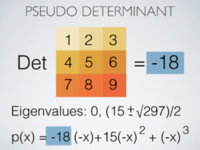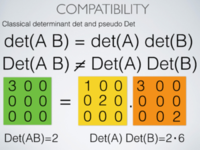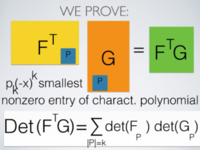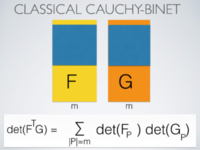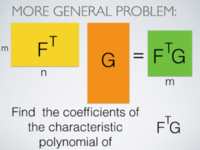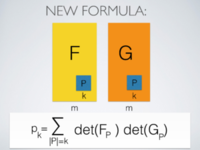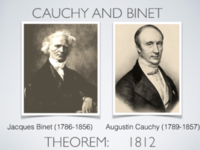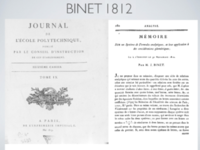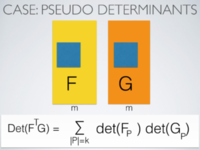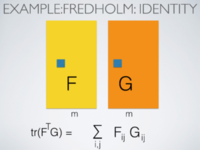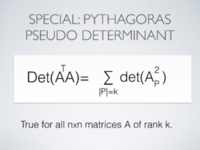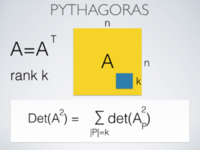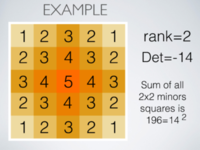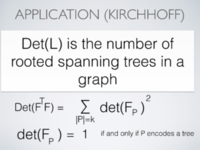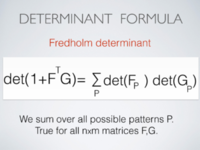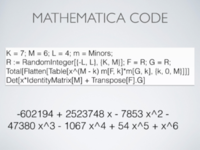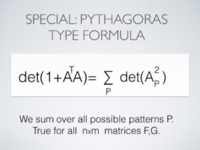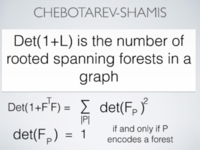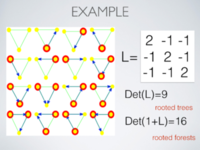July 23, 2014: The proofs of the article are in: O. Knill, Cauchy-Binet for pseudo-determinants Linear algebra and Applications (2014), Link. July 19, 2014:Having spent 14 years of my life with that problem (1987-2001) starting with undergraduate thesis and ending with a crash (which in a crucial postdoc time means certain academic death), I have vowed no more to touch "this ring". But lets like Bilbo in Rivendell try to see "that ring of ours" just for "one last time" ... It looks so simple: we want to estimate the determinants of (n x n) Jacobi matrices L of the form ```| V(1) 1 0 0 . ... 0 | | 1 V(2) 1 0 . ... 0 | | 0 1 V(2) 0 1 ... ... | | ... ... .... .... ... 0 | | 0 0 0 ...1 V(n-1) 1 | | 0 0 0 ....0 1 V(n) | , ``` where V(k) = 2 + c cos(x(k)) and where x(k) are generated by the Chirikov Standard map recursion x(k+1) - 2 x(k) + x(k-1) = c sin(x(k) and c is a parameter. By the Thouless formula, which conveniently follows from the Green-McKay-Weiss formula tr(An) -2 = det(L) for the transfer cocycle A of the discrete Schroedinger operator, which corresponds to the Jacobean of the Standard map, one only has to establish that det(L) grows exponentially with n. Actually log(det(L))/n converges to the Lyapunov exponent of the orbit, a limit which exists for almost all initial conditions (x(0),x(1)) by Oseledec's theorem. The conjecture is that if we average over all initial conditions on the two torus, we get a number which grows like log(c/2). Pesin theory shows that the number is important. It is the Sinai entropy of the map. But nobody has proven even for a single c, that the entropy is positive! And many talented mathematicians have worked on it. I thought already as an undergraduate that this was a mathematical scandal and went on a journey. By the way, there has been much progress since in the area of Lyapunov exponents, in particular by mathematicians like (Y.Sinai, L-S. Young, A. Gordon, B. Simon, M. Viana, S. Jitomirskaja, J.Last, J.Bourgain, A. Avila). Some lecture notes ov Viana on Lyapunov exponents can be found here. What makes the problem of Lyapunov exponents in the Standard map look so easy and tempting is that for other sequences x(k) like IID (or sufficiently decorrelated) random variables x(k) or having x(k)=x(k-1) + a almost periodic sequence, the problem can be tackled with: the random case has been covered by probability guys like Fuerstenberg or Kesten in the 60ies, the almost periodic case either with methods of Aubry (called Aubry duality argument) or Herman (sub-harmonicity estimate). The subharmonicity argument has been pushed further by Spencer,Sorets and others using the beautiful Jensen formula in complex analysis.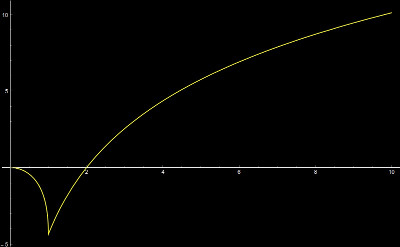I had myself tried with complex multivariable pluri-subharmonic arguments already as an undergraduate, then dived into methods from Jacobi matrices and my PhD thesis essentially dealt with searching for methods about that problem. Now, after proving the formula det(1+L K) = sumP det(L(P)) det(K(P)), where the right hand side is the sum over all minors, I of course started to think again about the Jacobi determinant problem. Here is an example of a line of thoughts (which does not need the full formula) as we take for K the identity matrix and take V(k) = 1 + c cos(x(k)) (we have removed 1 since the determinant formula again adds one). Now take for K the identity matrix and note that det(K(P)) is equal to 1 only if it is a diagonal minor and zero else. Now, we have, det(L) = sumP det(L(P)), where the right hand side is the sum over all diagonal minors of L. (this is Fredholm determinant picture of det(1+L). It is intriguing since the sum on the right hand side virtually only involves the statistics of the sequence x(k) and not their order (at least for most of the 2n minors). For the majority, the corresponding patterns matrices are diagonal. We only have non-diagonal matrices L(P) if the pattern P is such that two entries are adjacent. It would be Saruman's doing if not the main bulk of the contributions of the minors on the right hand side of Cauchy-Binet would not come from patterns which have not two adjacent entries and having a uniform distribution so we can control the expectation and relate it to the integral log(1+c cos(x)) from 0 to 2Pi. To the right, you see the graph of this integral as a function of c and thats positive for c>2 by Jensen. An other thing which comes in mind is to factor L=D2-1 (I did that in my thesis) and find det(1+L) = sumP det(DP)2. Here D is a Jacobi matrix again. But I'm not going to touch "Precious" again! ---- Even if I wants it ...

July 17, 2014: Here is the shortest code so far illustrating one of the theorems
```det(1+ATA) = sumP det(AP)^2
```
of the paper: every character was fought for in order to reduce it in size so that it became tweetable.
```M=5;A=RandomInteger[{-M,M},{M,M}];A=A+Transpose[A];
U=Flatten[Table[x^(M-k) Minors[A,k],{k,0,M}]];
{U.U,Det[x*IdentityMatrix[M]+A.A]}
```
More general is the following version, illustrating the paper's theorem which holds for any KxM matrices F,G:
```det(1+x FTG) = sumP x(M-|P|) det(FP) det(GP)
```
but it was with 158 characters no more tweetable:
```K=9;M=6;L=7; R:=RandomInteger[{-L,L},{K,M}];F=R;G=R;
U=Flatten[Table[x^(M-k) Minors[F,k]*Minors[G,k],{k,0,M}]];
{Total[U],Det[x*IdentityMatrix[M]+Transpose[F].G]}
```
[P.S. July 22: remove predefinitions and m=Minors hack makes Theorem 8 in the paper tweetable, here for 9x6 matrices taking values in [-7,7]
```R:=RandomInteger[{-7,7},{9,6}];F=R;G=R;m=Minors;
{Total[Flatten[Table[x^(6-k)m[F,k]*m[G,k],{k,0,6}]]],
Det[x*IdentityMatrix+Transpose[F].G]}
```
]

 By the way, here is an interesting power law behavior of the coefficients of the characteristic polynomial of the product FTG, compared with the behavior if we take FTF. One sees that the self correlation in the second case matters and that is obvious from our formula as we can write the coefficients as expectations of products of minors, which are independent if F and G are independent. ```K=60;M=73;L=10;R:=RandomInteger[{-L,L},{K, M}];F=R;G=R; V = Det[x*IdentityMatrix[M] - Transpose[F].F]; W = Det[x*IdentityMatrix[M] - Transpose[F].G]; ListPlot[Log[Abs[{CoefficientList[V,x],CoefficientList[W,x]}]]] ```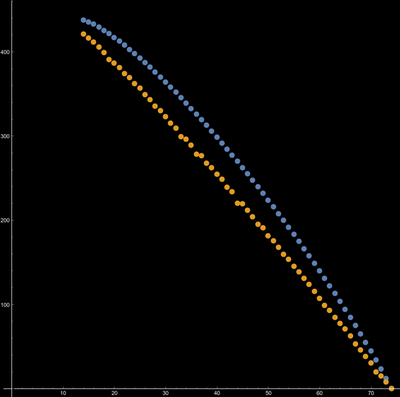The two mathematicians Binet and Cauchy lived around the same time, were in the same school, developed the same theorem at the same time and competed for the same position: they found the Cauchy-Binet formula at about the same time in 1812. More parallels: Binet graduated in 1806 from the Ecole Polytechnique, while Cauchy graduated from the same school in 1807. Their path crossed again in 1815, when Cauchy lost out to Binet for a chair at the Ecole polytechnique.Jacques Binet (1786-1856) Augustin Cauchy (1789-1857)

July 10, 2014: Here are the coordinates of the mathematicians of the beautiful matrix forest theorem (det(L+1) is the number of rooted forests): Elena Shamis was a PhD student of Pavel Chebotarev (from the Institute of Control Science). Shamis got her PhD in 1998. Chebotarev and Shamis are both descendants of Kolmogorov and Gelfand (the Gelfand of GNS construction or Gelfand-Naimark): (Andrei Kolmogorov - Israel Gelfand - Yakov Khurgin - Pavel Chebotarev -Elena Shamis).

July 6, 2014:
July 4, 2014:
• sign typo in the simplest calculus version of Cauchy Binet.
• added second identity Det(AT B) = Det(A BT) = Det(B AT) in 11) to have formally also the identity Det(AB)=Det(BA) for square matrices in the property list.

June 17, 2014: One major referee objection was that Pseudo determinants are not natural (i.e. its not a continuous functional). I spent this summer quite a bit of time revising the paper and also added a bit about Pseudo Pfaffians which I feel could have applications in differential geometry. The notations and definitions are now more natural and more consequences for classical determinants are more stressed.

Here is the Mathematica code for the Pseudo determinant:
```FirstNon0[s_]:=-(-1)^ArrayRules[s][[1,1,1]] ArrayRules[s][[1,2]];
PDet[A_]:=FirstNon0[CoefficientList[CharacteristicPolynomial[A,x],x]];
```
Here is the Pauli trace PTrace, the sum over patterns of size k:
```PTrace[A_,B_,k_]:=Module[{U,V,m=Length[A],n=Length[A[]],s={},t={}},
U=Partition[Flatten[Subsets[Range[m],{k,k}]],k]; u=Length[U];
V=Partition[Flatten[Subsets[Range[n],{k,k}]],k]; v=Length[V];
Do[s=Append[s,Table[A[[U[[i,u]],V[[j,v]]]],{u,k},{v,k}]],{i,u},{j,v}];
Do[t=Append[t,Table[B[[U[[i,u]],V[[j,v]]]],{u,k},{v,k}]],{i,u},{j,v}];
Sum[Det[s[[l]]]*Det[t[[l]]],{l,Length[s]}]];
```
One of the main results of the paper is the new identity for classical determinants and which holds for any nxm matrices F,G.
```det(1+z FTG) = Σ P zk(P) det(FP) det(GP)
```
where the sum to the right is over all patterns including empty patterns k(P)=0. It is a polynomial identity which is nice already for z=1. Here are some experiments:
```n=6; m=8; r=4; RandomMatrix[n_,m_]:=Table[RandomInteger[2r]-r,{n},{m}];
F=RandomMatrix[n,m]; G=RandomMatrix[n,m];
PTr[A_,B_]:=1+Sum[z^k PTrace[A,B,k],{k,1,Min[n,m]}];
{Det[IdentityMatrix[m]+z Transpose[F].G],PTr[F,G]}
```
For z=1, it immediately leads to the beautiful Chebotarev-Shamis theorem telling that the number of rooted forests in a graph is det(1+L) where L is the Laplacian. The proof of the theorem is comically simple when using multi-linear algebra. Here is an illustration how to work with exterior products of matrices in Mathematica: it shows the tautolocial lemma that the matrix product of the exterior product is the exterior product of the matrix product. A topic which unfortunately only recently made it into classical linear algebra texts. (As probably almost everybody else, I learned multi-linear algebra in the context of differential forms. For me personally, they are "in the blood" because I spent part of a 15 year eventually failed quest for establishing positive Lyapunov exponents in the standard map with multilinear methods).
```n=4; m=3; r=4; RandomMatrix[n_,m_]:=Table[RandomInteger[2r]-r,{n},{m}];
WedgeP[F_,k_]:=Module[{U,V,m=Length[F],n=Length[F[]]},
U=Partition[Flatten[Subsets[Range[m],{k,k}]],k];u=Length[U];
V=Partition[Flatten[Subsets[Range[n],{k,k}]],k];v=Length[V];
Table[Det[Table[F[[U[[i,u]],V[[j,v]]]],{u, k},{v, k}]],{i,u},{j,v}]];
F=RandomMatrix[n,m]; G=RandomMatrix[n,m];
{Transpose[WedgeP[F,2]].WedgeP[G,2],WedgeP[Transpose[F].G,2]}
```

1. June 1 2013: For (A)=0 => A=0, Det(A^-1)=1/Det(A) as well as stronger Pythagoras identity, the self-adjoint assumption was absent. This is incorporated in the ArXiv version.
2. June 10 2013: an updated version submitted to the ArXiv contains Mathematica code. See ArXiv version.

### Remarks since

1. June 15 2013: Pythagoras (Huppert-Willems call it Lagrange identity) would be obvious if one could see that the right hand side (the Pauli sum over all kxk minors) produce a number which is coordinate independent. But thats not clear a priori and only established by the proof in the exterior algebra.
2. It appears that the multi Fermion approach is not only the most elegant but also pedagogically the best approach to Cauchy-Binet. In the classical case, Shafarevich-Remizov call the approach "almost tautological". For a general linear algebra public, we present the proof via row reduction which already stretches what one can do in the classroom for a general student population (econ, life science, social science or engineering students and mostly non-math majors) My own handouts to that. Teaching a exterior algebra approach in such a one semester course (which features also linear and nonlinear differential equations including Fourier theory) is not quite realistic. But it can help a teacher to get more background insight. The usual elimination proof explains the multiplicativity a bit but seeing the determinant of a nxn matrix as a 1x1 matrix in a multiparticle picture helps to both to realize why the determinant is important and why nature likes Fermions: they are just natural.
3. June 18: The Pythagoras identity is called Lagrange identity in the linear algebra book of Huppert and Willems (German, 2. Edition, 2010). The alternating algebra seems have made it into textbook at first in Shafarevich and Remizov (2009). Having not been exposed to multilinear algebra in the intro linear algebra courses, I myself had learned this part of the "super symmetry" language and especially in the "Patodi" chapter of the book of Cycon-Kirsch-Froese-Simon.
4. June 22: we should have added this to the properties of Det: a nxn matrix A is nilpotent if and only if Det(A) = 0. Proof. By Cayley-Hamilton, a matrix is nilpotent if and only if the characteristic polynomial is pA(t) = tn which means all eigenvalues are 0. Also the PDet Mathematica code had to incorporate the nilpotent case. Looking at the first nonzero entry in the characteristic polynomial only gives the Det(A) if the first is not only the last nonzero entry.
5. June 27: had to mention Cauchy-Binet in the multivariable class as always when deriving the Lagrange identity |v|2 |w|2 - (v.w)2 = |v x w|2 which leads to |v x w| = |v| |w| sin(alpha) and immediately leads to the sin formula for a triangle after seeing it as twice the area of the triangle. The left hand side is Det(FT F) for the 3x2 matrix which contains v,w as columns. The right hand side is the Pauli sum. One of the course assistants, Steven Mackeret told me that the identity can also be seen as an identity for quaternions: if v=(a,b,c) and w=(p,q,r) and vxw = (s,t,u) then we look at the product of the quaternions V=a i + b j + c k, W = p i + q j + r k. Then V*W = -v.w + s i + t j + u k, So that |V*W|2 = |v x w|2 + (v.w)2 and |V|2 = |v|2, |W|2 = |w|2. The Cauchy-Binet identity here just tells that the quaternion length honors multiplication. Its historically interesting that cross and dot product were introduced together through quaternions.
6. June 28: sign typos in intro: the nilpotent matrix A2=0 of course gives Det(AT A)>0 but Det(A)2 =0.
7. July 5: A consequence of the main theorem is the beautiful formula
```det(1+FT G) = SumP det(FP) det(GP)
```
which is true for all nxm matrices F,G and which does not involve pseudo determinants at all. The right hand side sums over all possible minors with the assumption that the result is 1 if the pattern P is empty. Has somebody seen that? It immediately implies the "most important identity in Mathematics" det(1+FT G) = det(1+G FT) because both sides produce the same "dot product" on the right hand side. For self-adjoint matrices A, the identity implies
```det(1 + A2) = SumP det2(AP) ,
```
where the right hand side sums over all minors of A. This is useful for graphs because the right hand side counts the number of all trees, not necessarily maximal. If A is the Dirac operator, the left hand side is det(1+L). It is an other type of matrix tree theorem since the left hand side is the product of the shifted eigenvalues 1+lj of the Laplace-Beltrami operator on forms and the right hand side counts a signed sum of trees in a double cover of the simplex graph. Here is some Mathematica code which numerically explains the "Pauli dot product identity" mentioned above
```PTrace[A_,B_,k_]:=Module[{U,V,u,v,m=Length[A],n=Length[A[]],s={},t={}},
U=Partition[Flatten[Subsets[Range[m],{k,k}]],k]; u=Length[U];
V=Partition[Flatten[Subsets[Range[n],{k,k}]],k]; v=Length[V];
Do[s=Append[s,Table[A[[U[[i,u]],V[[j,v]]]],{u,k},{v,k}]],{i,u},{j,v}];
Do[t=Append[t,Table[B[[U[[i,u]],V[[j,v]]]],{u,k},{v,k}]],{i,u},{j,v}];
Sum[Det[s[[l]]]*Det[t[[l]]],{l,Length[s]}]];
PTraces[A_,B_]:=1+Sum[PTrace[A,B,k],{k,Min[n,m]}];
n=5; r=6; m=n; RandomMatrix[n_,m_]:=Table[RandomInteger[2r]-r,{n},{m}];
R:=Module[{},B=RandomMatrix[n,m]; B+Transpose[B]]; AA=R; BB=R;
{Det[IdentityMatrix[n] + Transpose[AA].BB], PTraces[AA,BB]}
```